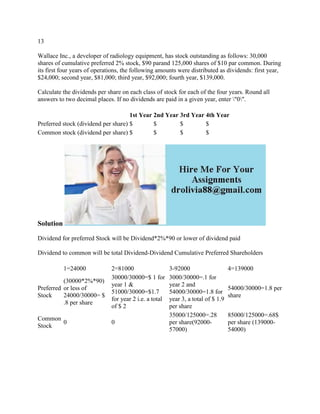Ce diaporama a bien été signalé.
Le téléchargement de votre SlideShare est en cours. ×

# 13 Wallace Inc-- a developer of radiology equipment- has stock outstan (1).docx

Publicité
Publicité
Publicité
Publicité
Publicité
Publicité
Publicité
Publicité
Publicité
Publicité
PublicitéChargement dans…3
×

## Consultez-les par la suite

1 sur 2 Publicité

# 13 Wallace Inc-- a developer of radiology equipment- has stock outstan (1).docx

13
Wallace Inc., a developer of radiology equipment, has stock outstanding as follows: 30,000 shares of cumulative preferred 2% stock, \$90 parand 125,000 shares of \$10 par common. During its first four years of operations, the following amounts were distributed as dividends: first year, \$24,000; second year, \$81,000; third year, \$92,000; fourth year, \$139,000.
Calculate the dividends per share on each class of stock for each of the four years. Round all answers to two decimal places. If no dividends are paid in a given year, enter \"0\".
Solution
Dividend for preferred Stock will be Dividend*2%*90 or lower of dividend paid
Dividend to common will be total Dividend-Dividend Cumulative Preferred Shareholders

.

13
Wallace Inc., a developer of radiology equipment, has stock outstanding as follows: 30,000 shares of cumulative preferred 2% stock, \$90 parand 125,000 shares of \$10 par common. During its first four years of operations, the following amounts were distributed as dividends: first year, \$24,000; second year, \$81,000; third year, \$92,000; fourth year, \$139,000.
Calculate the dividends per share on each class of stock for each of the four years. Round all answers to two decimal places. If no dividends are paid in a given year, enter \"0\".
Solution
Dividend for preferred Stock will be Dividend*2%*90 or lower of dividend paid
Dividend to common will be total Dividend-Dividend Cumulative Preferred Shareholders

.

Publicité
Publicité

### 13 Wallace Inc-- a developer of radiology equipment- has stock outstan (1).docx

1. 1. 13 Wallace Inc., a developer of radiology equipment, has stock outstanding as follows: 30,000 shares of cumulative preferred 2% stock, \$90 parand 125,000 shares of \$10 par common. During its first four years of operations, the following amounts were distributed as dividends: first year, \$24,000; second year, \$81,000; third year, \$92,000; fourth year, \$139,000. Calculate the dividends per share on each class of stock for each of the four years. Round all answers to two decimal places. If no dividends are paid in a given year, enter "0". 1st Year 2nd Year 3rd Year 4th Year Preferred stock (dividend per share) \$ \$ \$ \$ Common stock (dividend per share) \$ \$ \$ \$ Solution Dividend for preferred Stock will be Dividend*2%*90 or lower of dividend paid Dividend to common will be total Dividend-Dividend Cumulative Preferred Shareholders 1=24000 2=81000 3-92000 4=139000 Preferred Stock (30000*2%*90) or less of 24000/30000= \$ .8 per share 30000/30000=\$ 1 for year 1 & 51000/30000=\$1.7 for year 2 i.e. a total of \$ 2 3000/30000=.1 for year 2 and 54000/30000=1.8 for year 3, a total of \$ 1.9 per share 54000/30000=1.8 per share Common Stock 0 0 35000/125000=.28 per share(92000- 57000) 85000/125000=.68\$ per share (139000- 54000)